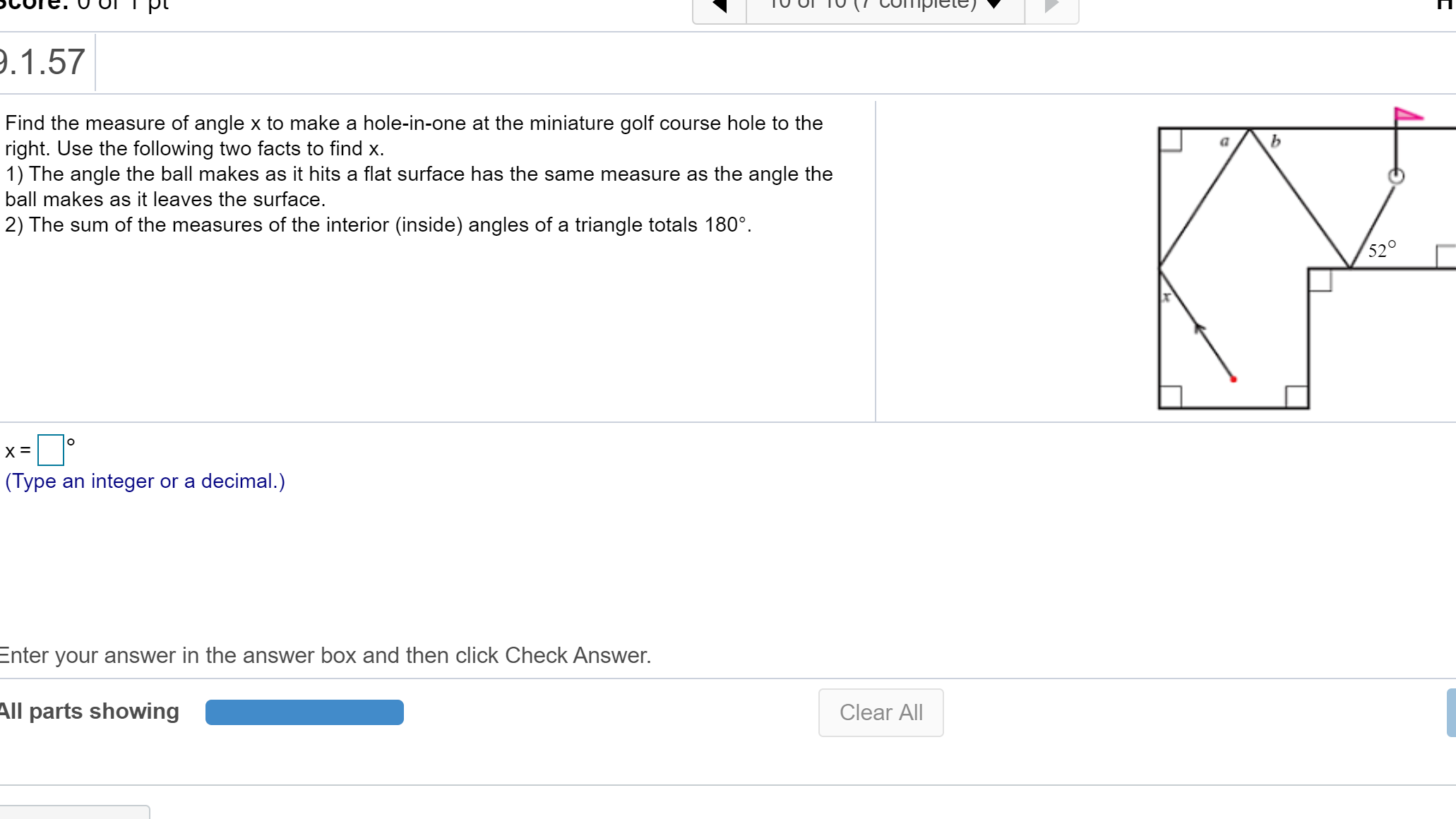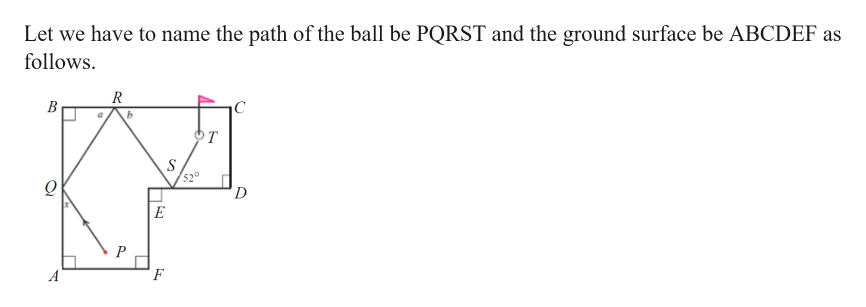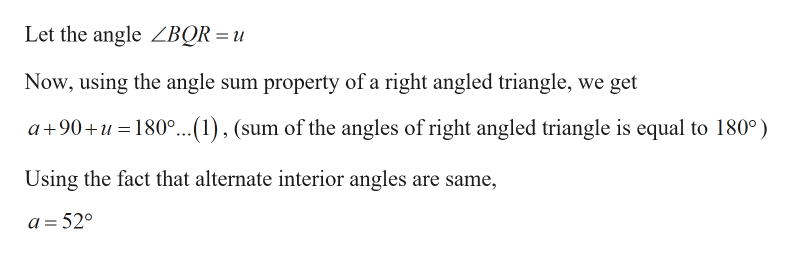# 9.1.57Find the measure of angle x to make a hole-in-one at the miniature golf course hole to theright. Use the following two facts to find x.1) The angle the ball makes as it hits a flat surface has the same measure as the angle theball makes as it leaves the surface.2) The sum of the measures of the interior (inside) angles of a triangle totals 180°.52°(Type an integer or a decimal.)Enter your answer in the answer box and then click Check Answer.All parts showingClear All

Question
6 viewshelp_outlineImage Transcriptionclose9.1.57 Find the measure of angle x to make a hole-in-one at the miniature golf course hole to the right. Use the following two facts to find x. 1) The angle the ball makes as it hits a flat surface has the same measure as the angle the ball makes as it leaves the surface. 2) The sum of the measures of the interior (inside) angles of a triangle totals 180°. 52° (Type an integer or a decimal.) Enter your answer in the answer box and then click Check Answer. All parts showing Clear All fullscreen
check_circle

Step 1

Given:

Step 2

Calculation:help_outlineImage TranscriptioncloseLet we have to name the path of the ball be PQRST and the ground surface be ABCDEF as follows В с b т S 520 D Е P F fullscreen
Step 3help_outlineImage TranscriptioncloseLet the angle ZBQR = u Now, using the angle sum property of a right angled triangle, we get a+90+u180..(1), (sum of the angles of right angled triangle is equal to 180°) Using the fact that alternate interior angles are same, a=520 fullscreen

### Want to see the full answer?

See Solution

#### Want to see this answer and more?

Solutions are written by subject experts who are available 24/7. Questions are typically answered within 1 hour.*

See Solution
*Response times may vary by subject and question.
Tagged in
MathGeometry

### Other